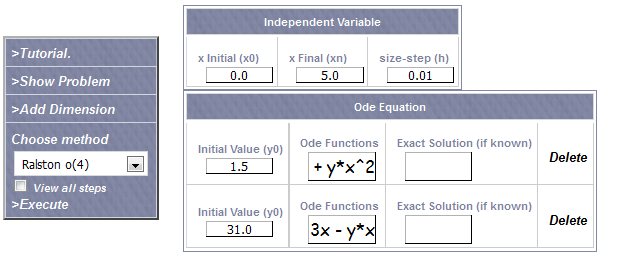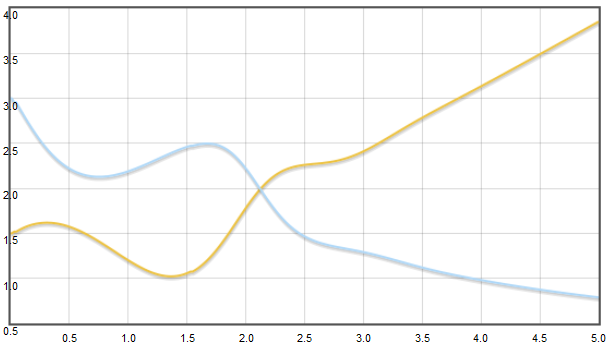# Brusselator: Non linear Odes system sample

The Brusselator is an oscillating problem for a certain type of chemical reactions modeled by a system of nonlinear differential equations

Consider the initial value problem

x' = 1-4x + yx2
y' = 3x - yx2

With
x(0) = 1.5
y(0) = 3.0

Let's use Runge-Kutta Calculator to solve numerically this probem.

If we introduce the problem in the application Runge-Kutta Calculator asIf we run the app Runge-Kutta Calculator obtain the graphNotice how the values x,y produce an oscillation before becoming unstable after a certain time

Run this with Runge-Kutta Calculator

# Was useful? want add anything?

Post here

### Post from other users

Post here